Porton, Victor

Restricting a reloid to lattice Gamma before converting it into a funcoid ★★

Author(s): Porton

Conjecture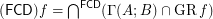for every reloid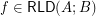.

Inner reloid through the lattice Gamma ★★

Author(s): Porton

Conjecture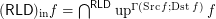for every funcoid.

Counter-example: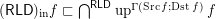for the funcoidis proved in this online article.

Keywords: filters; funcoids; inner reloid; reloids

Coatoms of the lattice of funcoids ★

Author(s): Porton

Problem   Let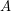and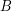be infinite sets. Characterize the set of all coatoms of the latticeof funcoids fromto. Particularly, is this set empty? Isa coatomic lattice? coatomistic lattice?

Keywords: atoms; coatoms; funcoids

Direct proof of a theorem about compact funcoids ★★

Author(s): Porton

Conjecture   Letis a-separable (the same as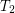for symmetric transitive) compact funcoid and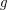is a uniform space (reflexive, symmetric, and transitive endoreloid) such that. Then.

The main purpose here is to find a direct proof of this conjecture. It seems that this conjecture can be derived from the well known theorem about existence of exactly one uniformity on a compact set. But that would be what I call an indirect proof, we need a direct proof instead.

The direct proof may be constructed by correcting all errors an omissions in this draft article.

Direct proof could be better because with it we would get a little more general statement like this:

Conjecture   Letbe a-separable compact reflexive symmetric funcoid andbe a reloid such that
\item; \item.

Then.

Generalized path-connectedness in proximity spaces ★★

Author(s): Porton

Letbe a proximity.

A setis connected regardingiff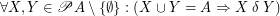.

Conjecture   The following statements are equivalent for every endofuncoidand a set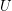:
\itemis connected regarding. \item For every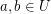there exists a totally ordered set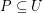such that,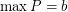, and for every partion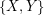ofinto two sets,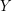such that, we have.

Keywords: connected; connectedness; proximity space

Every monovalued reloid is metamonovalued ★★

Author(s): Porton

Conjecture   Every monovalued reloid is metamonovalued.

Keywords: monovalued

Every metamonovalued reloid is monovalued ★★

Author(s): Porton

Conjecture   Every metamonovalued reloid is monovalued.

Keywords:

Every metamonovalued funcoid is monovalued ★★

Author(s): Porton

Conjecture   Every metamonovalued funcoid is monovalued.

The reverse is almost trivial: Every monovalued funcoid is metamonovalued.

Keywords: monovalued

Decomposition of completions of reloids ★★

Author(s): Porton

Conjecture   For composable reloidsandit holds
\item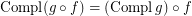ifis a co-complete reloid; \item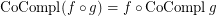ifis a complete reloid; \item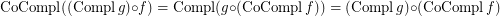; \item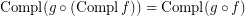; \item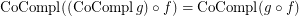.

Keywords: co-completion; completion; reloid

A construction of direct product in the category of continuous maps between endo-funcoids ★★★

Author(s): Porton

Consider the category of (proximally) continuous maps (entirely defined monovalued functions) between endo-funcoids.

Remind from my book that morphisms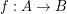of this category are defined by the formula(here and below by abuse of notation I equate functions with corresponding principal funcoids).

Letare endofuncoids,

We define(here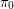andare cartesian projections).

Conjecture   The above defines categorical direct product (in the above mentioned category, with products of morphisms the same as in Set).

Keywords: categorical product; direct product